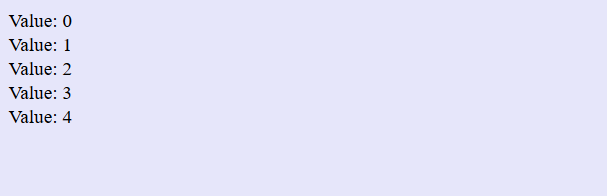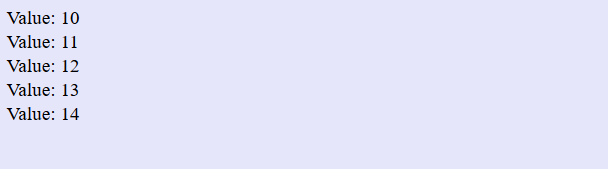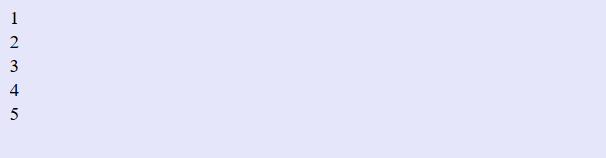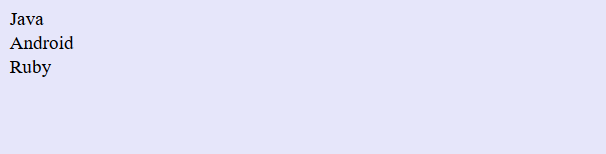# PHP Loops

## 07 Jul PHP Loops

Loops execute a block of code and this code executes while the condition is true. PHP Loops include while loop, do..while loop, for loop and foreach loop.

Let’s learn about PHP loops one by one. Here are the types of loops in PHP,

### PHP while Loop

In while loop, the block of code executes only when the condition is true. It executes as long as the condition is true.

Here’s the syntax:

#### Example

We’re running while loop from \$a = 0 to \$a <5 i.e. 5 iterations. When the value of a reaches 5, the iteration stops and the value gets printed as shown below,

Here’s the output,### PHP do…while loop

The block of code executes once, then the condition is checked, unlike while loop. The loop executes, till the condition is true.

Here’s the syntax:

#### Example

The code executes once with \$a = 10, then the condition \$a < 15 is checked. The loop executes till
\$a < 15,

Here’s the output,### PHP for loop

It is used when you can set the number of times a statement is to be executed.

Here’s the syntax:

The above syntax shows the following parameters,

• initialisation- The loop counter value initializes here.
• condition- If the condition is TRUE, the loop continues, else the loop ends.
• increment/decrement counter- Increase/ Decreases loop counter value

#### Example

Here you can see five iterations from \$a = 1 to \$a = 5,

Here’s the output,### PHP foreach loop

The foreach loop is used to loop through each key/value pair in an array. That means it only work for arrays.

Here’s the syntax:

The above syntax shows the following parameters,

• array- This is the array as represented by topics in the below example
• value-The array element value gets assigned to value.

#### Example

Here, we are printing the values of the given array topics:

Here’s the output,[email protected]

We work to create free tutorials for all.